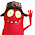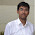# Multiplication of Two Large Numbers C Program

1. Multiplication operation of very large numbers in c language
2.How to get multiplication of two very large numbers larger or beyond than long int in c programming language
#include<stdio.h>
#include<math.h>
#include<stdlib.h>
#include<string.h>
#define MAX 10000
char * multiply(char [],char[]);
int main(){
char a[MAX];
char b[MAX];
char *c;
int la,lb;
int i;
printf("Enter the first number : ");
scanf("%s",a);
printf("Enter the second number : ");
scanf("%s",b);
printf("Multiplication of two numbers : ");
c = multiply(a,b);
printf("%s",c);
return 0;
}
char * multiply(char a[],char b[]){
static char mul[MAX];
char c[MAX];
char temp[MAX];
int la,lb;
int i,j,k=0,x=0,y;
long int r=0;
long sum = 0;
la=strlen(a)-1;
lb=strlen(b)-1;

for(i=0;i<=la;i++){
a[i] = a[i] - 48;
}
for(i=0;i<=lb;i++){
b[i] = b[i] - 48;
}
for(i=lb;i>=0;i--){
r=0;
for(j=la;j>=0;j--){
temp[k++] = (b[i]*a[j] + r)%10;
r = (b[i]*a[j]+r)/10;
}
temp[k++] = r;
x++;
for(y = 0;y<x;y++){
temp[k++] = 0;
}
}

k=0;
r=0;
for(i=0;i<la+lb+2;i++){
sum =0;
y=0;
for(j=1;j<=lb+1;j++){
if(i <= la+j){
sum = sum + temp[y+i];
}
y += j + la + 1;
}
c[k++] = (sum+r) %10;
r = (sum+r)/10;
}
c[k] = r;
j=0;
for(i=k-1;i>=0;i--){
mul[j++]=c[i] + 48;
}
mul[j]='\0';
return mul;
}
Sample output of above code:
Enter the first number: 55555555
Enter the second number: 3333333333 Multiplication of two numbers:
185185183314814815
Logic for multiplication of large numbers
As we know in c there are not any such data types which can store a very large numbers.  For example we want to solve the expression:
55555555 * 3333333333

Result of above expression is very big number which beyond the range of even long int or long double.  Then question is how to store such a big numbers in c?

Solution is very simple i.e. using array. Above program has used same logic that is we are using as usual logic to multiply two numbers except instead of storing the data in the normal variables we are storing into the array.

1.Totally free program for learning multiplication tables for children.
http://Aztekium.pl/Multiplication/

- Very helpful for math teachers!

- A teacher from a distance sees who knows multiplication!
- A child can learn math at home
- A child can use a mobile phone
2.